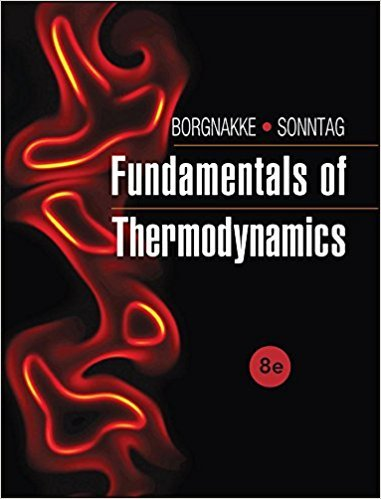×
Get Full Access to Fundamentals Of Thermodynamcs - 8 Edition - Chapter 7 - Problem 155hp
Get Full Access to Fundamentals Of Thermodynamcs - 8 Edition - Chapter 7 - Problem 155hp

×

# Air flows into an insulated nozzle at 1 MPa, 1200 K withISBN: 9781118131992 58

## Solution for problem 155HP Chapter 7

Fundamentals of Thermodynamcs | 8th Edition

• Textbook Solutions
• 2901 Step-by-step solutions solved by professors and subject experts
• Get 24/7 help from StudySoup virtual teaching assistantsFundamentals of Thermodynamcs | 8th Edition

4 5 1 282 Reviews
10
0
Problem 155HP

Problem 155HP

Air flows into an insulated nozzle at 1 MPa, 1200 K with 15 m/s and a mass flow rate of 2 kg/s. It expands to 650 kPa, and the exit temperature is 1100 K. Find the exit velocity and the nozzle efficiency.

Step-by-Step Solution:
Step 1 of 3

Step 2 of 3

Step 3 of 3

##### ISBN: 9781118131992

Fundamentals of Thermodynamcs was written by and is associated to the ISBN: 9781118131992. Since the solution to 155HP from 7 chapter was answered, more than 249 students have viewed the full step-by-step answer. This full solution covers the following key subjects: exit, nozzle, insulated, expands, Find. This expansive textbook survival guide covers 7 chapters, and 1462 solutions. The answer to “Air flows into an insulated nozzle at 1 MPa, 1200 K with 15 m/s and a mass flow rate of 2 kg/s. It expands to 650 kPa, and the exit temperature is 1100 K. Find the exit velocity and the nozzle efficiency.” is broken down into a number of easy to follow steps, and 42 words. This textbook survival guide was created for the textbook: Fundamentals of Thermodynamcs , edition: 8. The full step-by-step solution to problem: 155HP from chapter: 7 was answered by , our top Engineering and Tech solution expert on 08/03/17, 05:05AM.

Unlock Textbook Solution